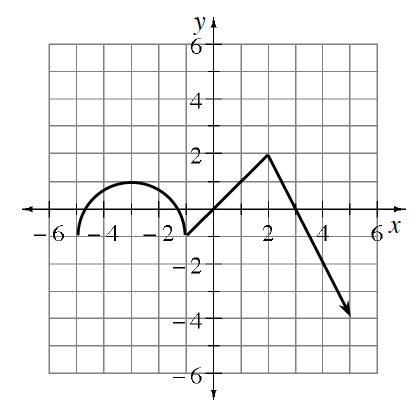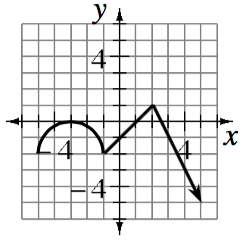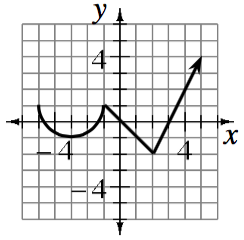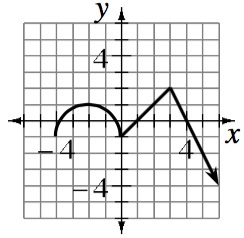### Home > INT2 > Chapter 9 > Lesson 9.4.1 > Problem9-141

9-141.

Copy the graph of $y = f(x)$ at right onto graph paper, and then graph each of the following transformations.

1. $y = f(x) - 1$

2. $y = -f(x)$

3. $y = f(x - 1)$This transformation is a translation along the $y$ axis. Piecewise graph with 3 connected sections First: semicircle, passing through the points (negative 5, comma negative 2), (negative 3, comma 0), & (negative 1, comma negative 2). Second: Segment from the point (negative 1, comma negative 2), to the point (2, comma 1). Third: Ray from the point (2, comma 1), passing through the point (5, comma negative 5).

A negative coefficient in front of $f(x)$ means the graph needs to be reflected over the $x$ axis. Piecewise graph with 3 connected sections First: semicircle, passing through the points (negative 5, comma 1), (negative 3, comma negative 1), & (negative 1, comma 1). Second: Segment from the point (negative 1, comma 1), to the point (2, comma negative 2). Third: Ray from the point (2, comma negative 2), passing through the point (5, comma 4).

This transformation is a translation along the $x$ axis. Piecewise graph with 3 connected sections First: semicircle, passing through the points (negative 4, comma negative 1), (negative 2, comma 1), & (0, comma negative 1). Second: Segment from the point (0, comma negative 1), to the point 3, comma 2). Third: Ray from the point (3, comma 2), passing through the point (6, comma negative 4).